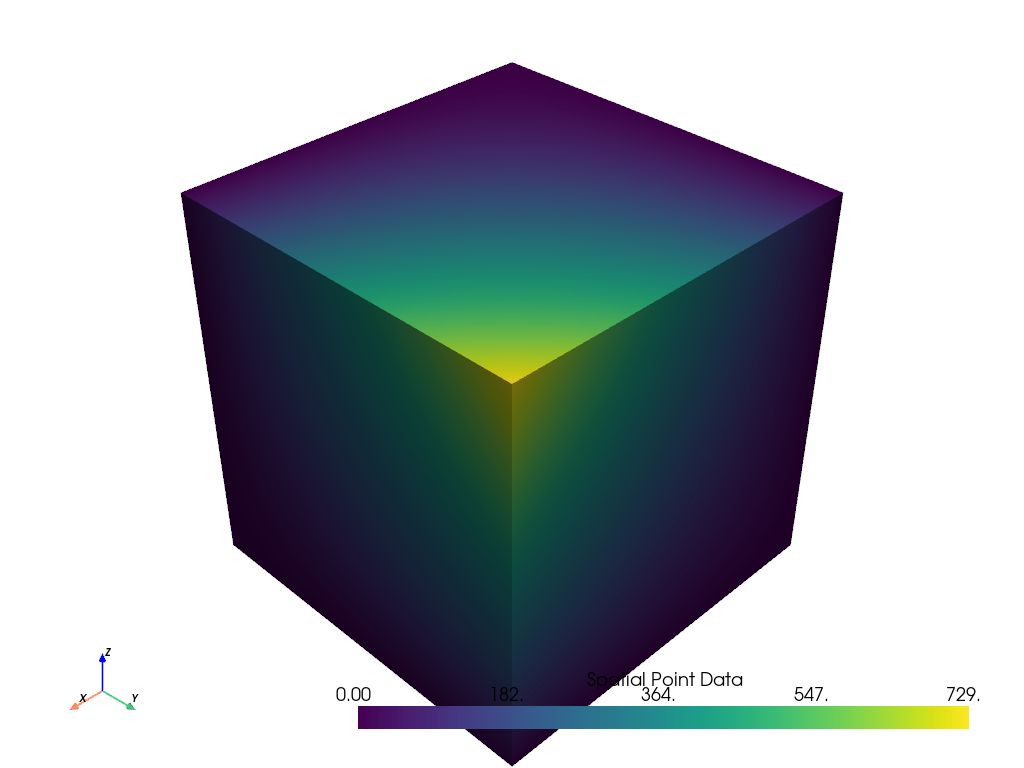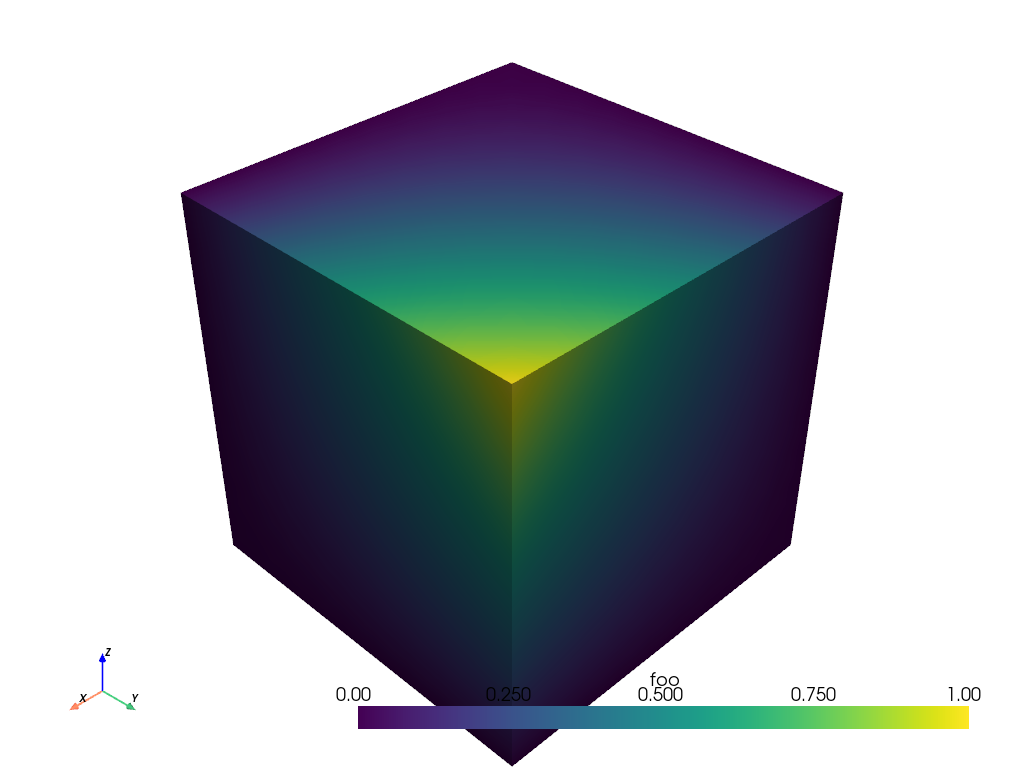# Normalize Array¶

This example will demonstrate how to perform a normalization or any custom mathematical operation on a single data array for an input data set.

This filter allow the user to select an array from the input data set to be normalized. The filter will append another array to that data set for the output. The user can specify how they want to rename the array, can choose a multiplier, and can choose from two types of common normalizations: Feature Scaling and Standard Score.

This example demos `PVGeo.filters.NormalizeArray`

```import numpy as np
import pyvista
from pyvista import examples
import PVGeo
from PVGeo.filters import NormalizeArray
```

Create some input data. this can be any vtkDataObject

```mesh = examples.load_uniform()
title = 'Spatial Point Data'
mesh.plot(scalars=title)
```Out:

```[(21.886664873203234, 21.886664873203234, 21.886664873203234),
(4.5, 4.5, 4.5),
(0.0, 0.0, 1.0)]
```
```# Apply the filter
f = NormalizeArray(normalization='feature_scale', new_name='foo')
output = f.apply(mesh, title)
print(output)
```

Out:

```UniformGrid (0x7f6a5d625d08)
N Cells:      729
N Points:     1000
X Bounds:     0.000e+00, 9.000e+00
Y Bounds:     0.000e+00, 9.000e+00
Z Bounds:     0.000e+00, 9.000e+00
Dimensions:   10, 10, 10
Spacing:      1.000e+00, 1.000e+00, 1.000e+00
N Arrays:     3
```
```output.plot(scalars='foo')
```Out:

```[(21.886664873203234, 21.886664873203234, 21.886664873203234),
(4.5, 4.5, 4.5),
(0.0, 0.0, 1.0)]
```

Total running time of the script: ( 0 minutes 1.576 seconds)

Gallery generated by Sphinx-Gallery